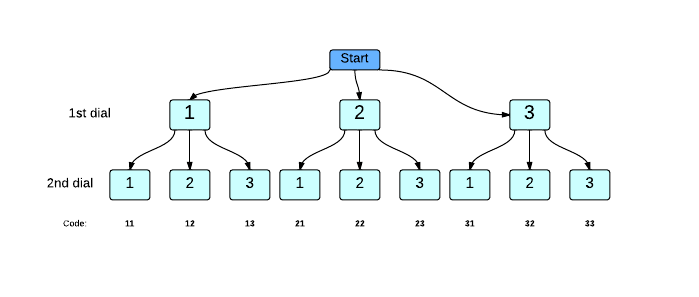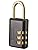# Permutations with Repetition

There are 2 kinds of permutations:

• Permutations with Repetition - You can re-use the same element within the order, such as in the lock from the previous question, where the code could be "000".

• Permutations without repetition - Each element can only appear once in the order. For example, on some locks to houses, each number can only be used once. Similarly, when you're ranking people in the poetry contest, each slot needs to be given to a different person.

Calculating Permutations with Repetition
Here's a question to ponder: Let's say you had a simple lock like with just 2 dials and 3 digits per dial. A code consists of 2 digits in the correct order. How many possible codes could it have?(Scroll down for the solution.)

Permutation Tree
This tree plots out the different possibile codes for the lock:For each of the 3 possibilities of the first dial, there are 3 possibilities for the second dial. This means there will be 3x3 = 9 possibilities in total. Whenever there are n ways of doing one thing and m ways of doing another, there are n * m ways of doing both together.

So if you added a 3rd dial, then there would be 9*3 = 27 possible permutations of all 3 dials. This is because for each of the 9 permutations of the 1st two dials, there will be 3 possibilities for the 3rd dial.

Formula for Permutations with Repetition
If there are N possibilities for each element, and there are E elements, then:
Total # of Possibilities = N * N * N * ... (E times)
This can also be written as an exponent, as NE.### Challenge

How many codes are possible for the 3-dial lock with 10 digits (0-9) on each dial? (Enter a number answer.)

Alternatively, you can try out Learneroo before signing up.

•#### Chillex

I got a little confused about N as possibilities and E as Dials. But I still got the correct answer.

•#### rodrigo perez

There are 10 ways to set each of the 3 dials... so the first combination would be 000, and the last one, 999.

•#### Ian Rainford

why is 103 incorrect?

•#### Learneroo

@Ian, in this question you need to enter the actual answer.